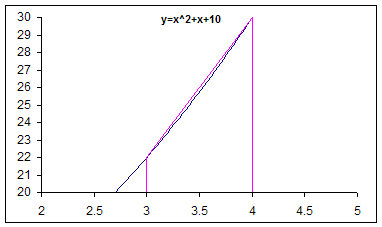You are on the Home/Excel/Tutorials/Integration pageShare Your

Keywords: Numerical Integration Simpson Trapezoid Approximation

Introduction

Numerical Integration

Trapezoid Rule

Simpson's Rule

Simpson's 1/3 Rule

Implementation in Excel

Using Named Formulas

Using VBA

Numerical Integration

Trapezoid RuleThe area of the trapezoid defined by the pink lines above is given by

Simpson's Rule

Simpson's 1/3 Rule